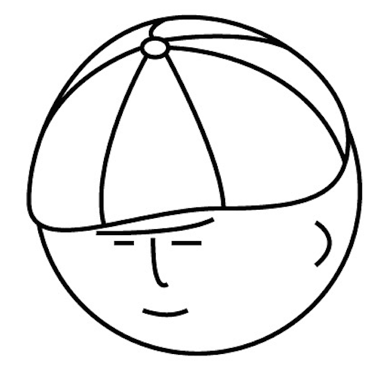# マツシタのお勉強メモ

#### マツシタのお勉強

##### welcome to my engineering blog
###### Hacker Rank# Problem

Write an algorithm to find the `next` node (i.e., in-oder successor) of a given node in a binary search tree. You may assume that each node has a link to its parent.

# Solution

This problem can be solved by thinking the two patterns. First pattern is in case the given node has right node, and second pattern is in case the given node dose not have right node.

#### the node has right node

In this case, the next node can be calculated by the following steps.

1. traverse right node once.
2. traverse left node until the left node is null
3. when the loop is broken, the node is next node.

This traversal is to bottom.

#### the node dose not have right node

In this case, the next node can be calculated by the following steps.

1. traverse the parent node until the left node of the parent node dose not coincide the current node.
2. when the above loop is broken, the node is next node.

This traversal is to up.